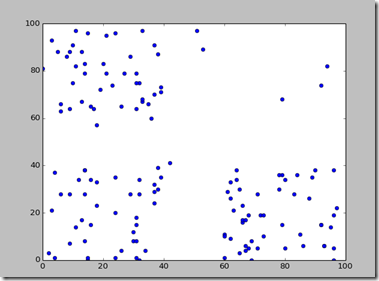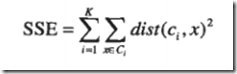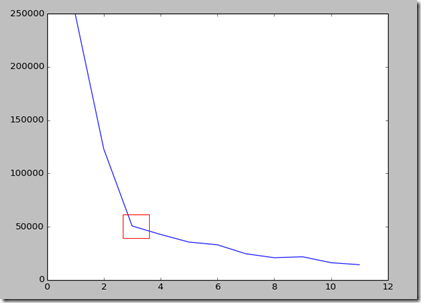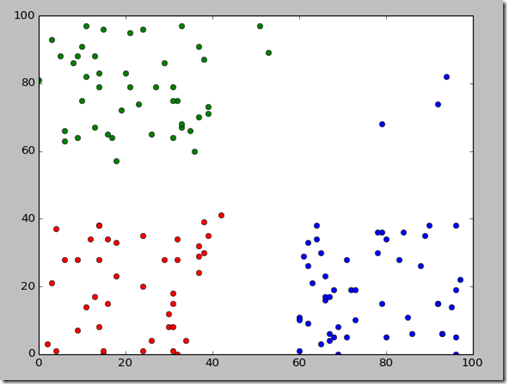# 聚类分析的K均值算法(Python实现)

2014/03/18 13:49

K均值用于n维空间中的对象，它只需要对象之间的临近性度量，例如使用欧几里德距离，可以用于广泛的数据，但是它属于不稳定的算法。

matplotlib的安装详见博客：http://my.oschina.net/bery/blog/203595

``````

import

numpy as np

import

pylab as pl

x, y

=

'

2.csv

'

, delimiter

=

'

,

'

, unpack

=

True)

pl.plot(x, y,

'

o

'

)
pl.show()

````````````

#

计算SSE

def

calc_sse(x, y, k_count):
count

=

len(x)

#

点的个数

k

=

rd.sample(range(count), k_count)

#

随机选择K个点

k_point

=

[[x[i], [y[i]]]

for

i

in

k]
k_point.sort()

#

保证有序

sse

=

[[]

for

i

in

range(k_count)]

while

True:
ka

=

[[]

for

i

in

range(k_count)]

#

存储每个簇的索引

sse

=

[[]

for

i

in

range(k_count)]

#

遍历所有点

for

i

in

range(count):
cp

=

[x[i], y[i]]

#

当前点

#

计算cp点到所有质心的距离

_sse

=

[distance(k_point[j], cp)

for

j

in

range(k_count)]

#

cp点到那个质心最近

min_index

=

_sse.index(min(_sse))

#

把cp点并入第i簇

ka[min_index].append(i)
sse[min_index].append(min(_sse))

#

更换质心

k_new

=

[]

for

i

in

range(k_count):
_x

=

sum([x[j]

for

j

in

ka[i]])

/

len(ka[i])
_y

=

sum([y[j]

for

j

in

ka[i]])

/

len(ka[i])
k_new.append([_x, _y])

k_new.sort()

#

排序

#

更换质心

if

(k_new

!=

k_point):
k_point

=

k_new

else

:

break

s

=

0

for

i

in

range(k_count):
s

+=

sum(sse[i])

return

s

``````

SSE曲线的结果显示如下：``````

#

K均值算法

def

k_means(x, y, k_count):
count

=

len(x)

#

点的个数

#

随机选择K个点

k

=

rd.sample(range(count), k_count)

k_point

=

[[x[i], [y[i]]]

for

i

in

k]

#

保证有序

k_point.sort()

while

True:
km

=

[[]

for

i

in

range(k_count)]

#

存储每个簇的索引

#

遍历所有点

for

i

in

range(count):
cp

=

[x[i], y[i]]

#

当前点

#

计算cp点到所有质心的距离

_sse

=

[distance(k_point[j], cp)

for

j

in

range(k_count)]

#

cp点到那个质心最近

min_index

=

_sse.index(min(_sse))

#

把cp点并入第i簇

km[min_index].append(i)

#

更换质心

k_new

=

[]

for

i

in

range(k_count):
_x

=

sum([x[j]

for

j

in

km[i]])

/

len(km[i])
_y

=

sum([y[j]

for

j

in

km[i]])

/

len(km[i])
k_new.append([_x, _y])

k_new.sort()

#

排序

if

(k_new

!=

k_point):
k_point

=

k_new

else

:

return

km

``````http://pan.baidu.com/s/1c05pcQW

1
7 收藏

### 作者的其它热门文章2018/12/14 10:02

1 评论
7 收藏
1